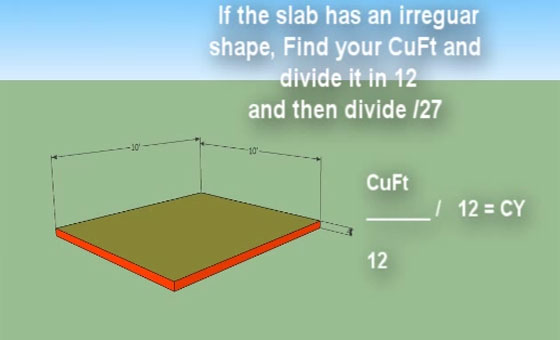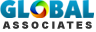# Some useful guidance to estimate concrete for any projectIn order to work out how much concrete is required for any particular area, initially one should ensure that the concrete is ordered in cubic yards otherwise CuYards.

The conversion amongst Cuft and Cuyds is described with the constant (27).

Go through the following simple processes to accomplish it :-

• At first figure out the quantity of cubic feet you comprise in the area of concrete to be estimated by applying the formula: width x length x dept = cubic feet.
• Afterward, partition the cubic feet with the constant 27 and that will bring about the cubic yards necessary to stuff a set area.

Use the following quick tricks to estimate concrete when depth is already defined:

For pouring a 4" thick slab:

Multiply width by length and divide the sequential number with the constant (80).
Ej.,
(20'x40') = 800sqft / 80 = 10 Cu Yards of concrete!
For pouring a 6" thick slab:

Multiply width by length and divide the sequential number with the constant (50).
Ej.,
(20'x40') = 800sqft / 50 = 16 Cu Yards of concrete!
General Formula:

Multiply width by length by depth and divide it with the constant (27).
Ej.,
(20'x40'x1'6")=1200Cuft. /27= 44.44 Cu yards or 44 ½ yards. Trick: Opt for ordering 1/4 yard more for each 50 ft of hose while utilizing a concrete pump.

With the above mentioned formulas, one can easily estimate any size pad of concrete.

Watch the online demo.# Takeoff Tools